# Self Induction and Self Inductance and Derivation of Inductance

## Self Induction

Self induction is a phenomenon by which a changing electric current produces an induced emf across the coil itself.

## Self Inductance

Self inductance is the ratio of induced electromotive force (EMF) across a coil to the rate of change of current through the coil. We denote self inductance or coefficient of with English letter L. Its unit is Henry (H).
Since, the induced emf (E) is proportional to the current changing rate, we can write,But the actual equation is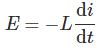Why there is Minus (-) sign?
According to Lenz’s Law, the induced emf opposes the direction of the rate of change of current. So their value is same but sign differs.

## Derivation of Inductance

For the DC source, when the switch is ON, i.e. just at t = 0+, a current starts flowing from its zero value to a certain value and with respect to time, there will be a rate of change in current momentarily. This current produces changing flux (φ) through the coil. As current changes flux (φ) also changes and the rate of change with respect to the time is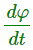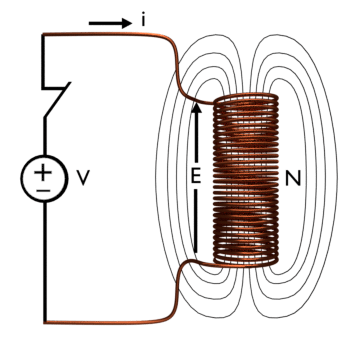Now by apply Faraday’s Law of Electromagnetic Induction, we get,Where, N is the number of turn of the coil and e is the induced EMF across this coil.
Considering Lenz’s law we can write the above equation as,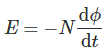Now, we can modify this equation to calculate the value of inductance.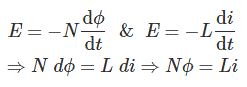So,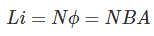[B is the flux density i.e. B =φ/A, A is area of the coil],
[Nφ or Li is called magnetic flux Linkage and it is denoted by Ѱ]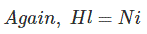Where H is the magnetizing force due to which magnetic flux lines flow from south to north pole inside the coil, l (small L) is the effective length of the coil and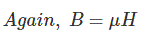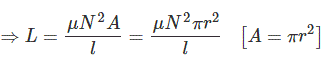r is the radius of the coil cross-sectional area.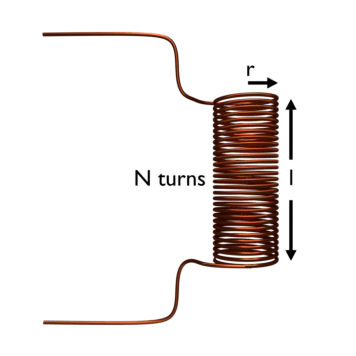Self inductance, L is a geometric quantity; it depends only on the dimensions of the solenoid, and the number of turns in the solenoid. Furthermore, in a DC circuit when the switch is just closed, then only momentarily effect of self-inductance occurs in the coil. After some time, no effect of self inductance remains in the coil because after certain time the current becomes steady.

But in AC circuit, the alternating effect of current always causes the self-induction in the coil, and a certain value of this self-inductance gives the inductive reactance (XL = 2πfL) depending on the value of supply frequency.

### Video Presentation of Self Inductance

Want To Learn Faster? 🎓
Get electrical articles delivered to your inbox every week.
No credit card required—it’s 100% free.